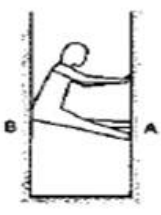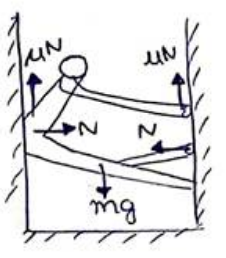# Solve the following :

Question:

A person $(40 \mathrm{~kg})$ is managing to be at rest between two vertical walls by pressing one wall A by his hands and feet and the other wall Bby his back. Assume that the friction coefficient between his body and the walls is $0.8$ and that limiting friction acts at all the contacts.

(a) Show that the person pushes the two walls with equal force.

(b) Find the normal force exerted by either wall on the person. Take $g=10 \mathrm{~m} / \mathrm{s}^{2}$.Solution:(a) Since the man is at rest horizontally. So, both by both walls on man will be equal and opposite in direction.

(b) In vertical equilibrium condition

$2 \mu N=m g$

$2 \times 0.8 \times N=40 \times 10$

$N=250 \mathrm{~N}$